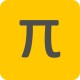# Spot the difference.

I’m in the process of migrating the sample code for using the LiDAR-Lite to the format I normally use.  It’s not been as simple as I thought.  I’ve narrowed the problem down to this.  Look for the SPOT THE DIFFERENCE comments.

```from __future__ import division
import smbus
import time

class Lidar_Lite:

__GLL_ACQ_COMMAND       = 0x00
__GLL_STATUS            = 0x01
__GLL_SIG_COUNT_VAL     = 0x02
__GLL_ACQ_CONFIG_REG    = 0x04
__GLL_VELOCITY          = 0x09
__GLL_PEAK_CORR         = 0x0C
__GLL_NOISE_PEAK        = 0x0D
__GLL_SIGNAL_STRENGTH   = 0x0E
__GLL_FULL_DELAY_HIGH   = 0x8F
__GLL_FULL_DELAY_LOW    = 0x10
__GLL_OUTER_LOOP_COUNT  = 0x11
__GLL_REF_COUNT_VAL     = 0x12
__GLL_LAST_DELAY_HIGH   = 0x14
__GLL_LAST_DELAY_LOW    = 0x15
__GLL_UNIT_ID_HIGH      = 0x16
__GLL_UNIT_ID_LOW       = 0x17
__GLL_I2C_ID_HIGHT      = 0x18
__GLL_I2C_ID_LOW        = 0x19
__GLL_THRESHOLD_BYPASS  = 0x1C
__GLL_I2C_CONFIG        = 0x1E
__GLL_ACQ_COMMAND       = 0x40
__GLL_MEASURE_DELAY     = 0x45
__GLL_PEAK_BCK          = 0x4C
__GLL_CORR_DATA         = 0x52
__GLL_CORR_DATA_SIGN    = 0x53
__GLL_ACQ_SETTINGS      = 0x5D
__GLL_POWER_CONTROL     = 0x65

def __init__(self):
self.distWriteReg = 0x00
self.distWriteVal = 0x04

try:
self.bus = smbus.SMBus(1)
except:
return

############################ SPOT THE DIFFERENCE ###########################
############################ SPOT THE DIFFERENCE ###########################

def getDistance(self):
return ((dist1 << 8) + dist2) / 100

def getVelocity(self):
return (self.signedInt(vel)) / 100

def signedInt(self, value):
return value if value < 128 else (value - 256)

ll = Lidar_Lite()

start_time = time.time()
ii = 0
while ii < 100:
ii += 1
velocity = ll.getVelocity()
distance = ll.getDistance()
print "Velocity %f; Distance %f" % (velocity, distance)
time.sleep(0.1)
print "%d reads took %f seconds" % (ii, time.time() - start_time)
```

Using the commented out line, and all works.
Using the uncommented line, and it fails.

The register sets whether data is updated automatically rather than when a command is set to get a new reading.

There’s no python error; neither raise Python syntax errors.

Anyone got any ideas as to what the problem could be?

## 2 thoughts on “Spot the difference.”

1.Jeremy on said:

“Using the uncommented line, and it fails.” – what constitutes a fail? What kind of output do you get when there is a failure?

•Hove on said:

The line above the “Spot the Difference” sets it to permanently loop sampling after the first command to the LiDAR-Lite to take a sample.

The “SPOT THE DIFFERENCE” lines are that command to take a sample. Using the upper one, I get different distance readings as I move a MagPi magazine towards / away from the LiDAR-Lite. Using the lower one, I get no change in the output value – it’s like a single reading that’s being returned repeatedly for each read of the height regardless of whether that distance has changes.

The only real difference is how the registers are defined – one just inside the class definition and the other inside the __init__() function. There’s something subtle about how I’ve defined __GLL_ACQ_COMMAND that’s wrong somehow.

This site uses Akismet to reduce spam. Learn how your comment data is processed.